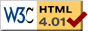### Journée-séminaire de combinatoire

#### (équipe CALIN du LIPN, université Paris-Nord, Villetaneuse)

Le 09 décembre 2008 à 14h en B107, Flavia Bonomo nous parlera de : On variations of the graph coloring problem

Résumé : A coloring of a graph is an assignment of natural numbers to its vertices in such a way that adjacent vertices receive different colors. This well-known problem is a basic model for frequency assignment and resource allocation problems. In order to take into account particular constraints arising in practical settings, more elaborate models of vertex coloring have been defined in the literature. One of such generalized models is the list-coloring problem, which considers a prespecified set of available colors for each vertex. The list-coloring problem is NP-complete even for split graphs, interval graphs, and bipartite graphs. We consider some particular cases of the list-coloring problem: μ-coloring, where each vertex has its own upper bound for the color to be assigned, and (γ,μ)-coloring, where each vertex has its own lower and upper bounds for the color to be assigned. The μ-coloring problem arises in the context of classroom allocation to courses, where each course must be assigned a classroom which is large enough so it fits the students taking the course. We will present some polynomial time algorithms to solve these problems on classes where list-coloring is NP-complete.

 Dernière modification : Monday 24 January 2022Contact pour cette page : Cyril.Banderier at lipn.univ-paris13.fr